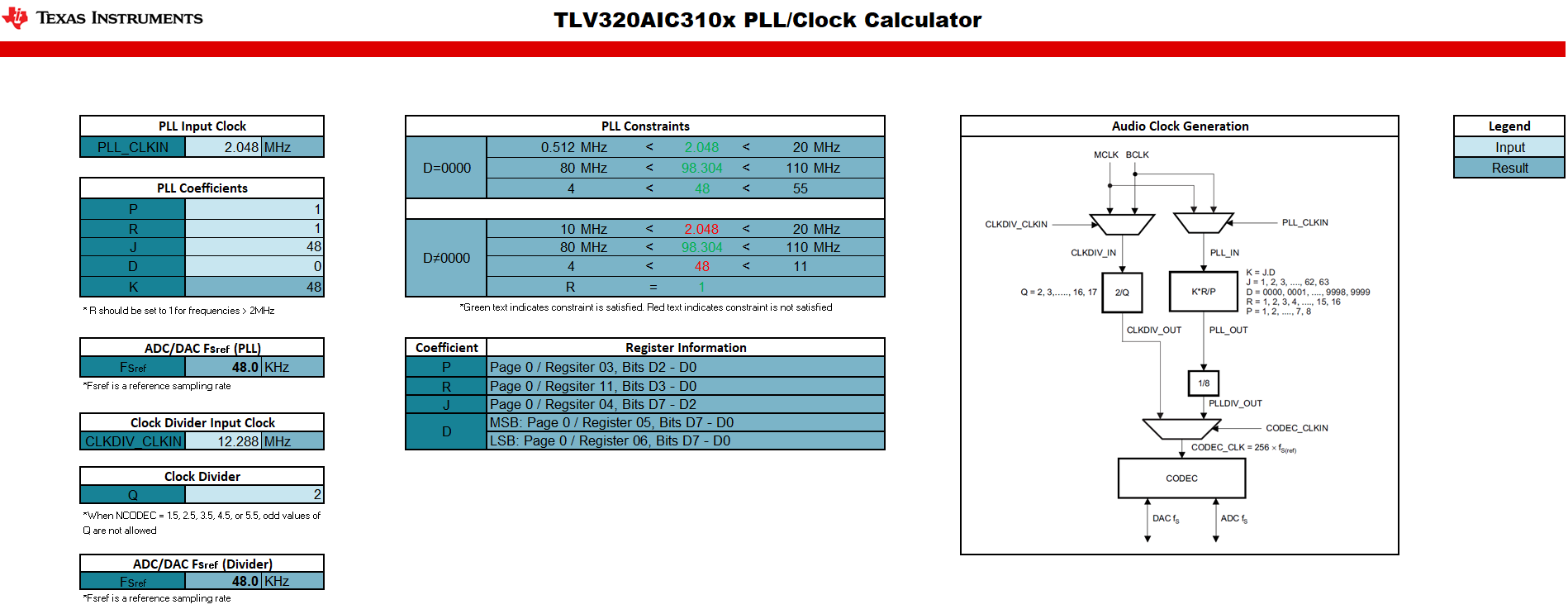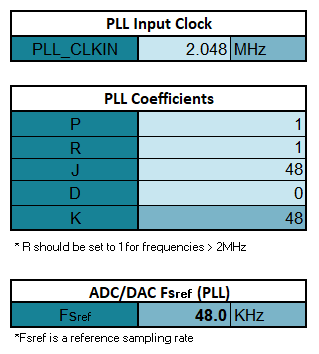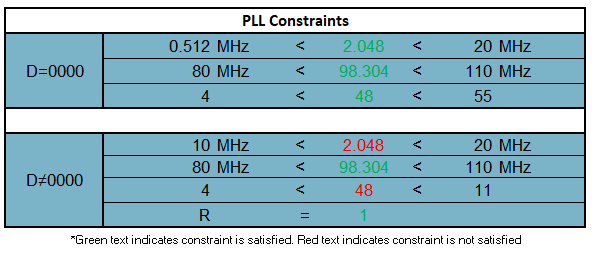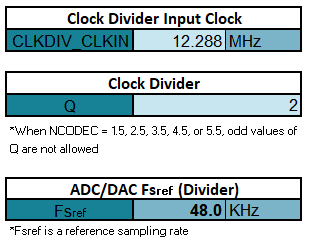• Resolved

# [FAQ] I need help configuring the PLL on my audio CODEC/ADC. Is there an easy way to do so?

Hello,

Configuring the PLL and clocks on the audio CODEC/ADC is a bit confusing to me. Is there an easy way to get or confirm the coefficients I need? I am using an input clock of 2.048MHz and wish to run at an Fs of 48kHz.

Thanks,

Aaron

• Hi,

Configuring the PLL or clocking scheme for our audio CODECs and ADCs can seem like a daunting task. Since the PLL is designed to accept a wide range of input clocks, both common and uncommon, there are many ways to configure it and one can easily get lost doing so. This is why we have a PLL CODEC/ADC Calculator that helps you confirm your PLL configuration!

The Audio CODEC/ADC PLL Calculator can be found here.

To get started with this tool, there is a Table of Contents sheet that provides links to families of devices and their respective PLL/Clock calculator. You may simply select the family you have and it will take you to the proper calculator to use. As an example, if you select the TLV320AIC310x link, it will take you to the following sheet:Once here you can start inputting different PLL coefficients or check the ones you have already generated! There are different blocks inside this sheet that allow you to input information as well as blocks that provide values such as Fsref and check constraints.

If you are providing an input clock of 2.048MHz, you can enter this value in the PLL Input Clock block. The next step is to then change the P, R, J and D coefficients found in the PLL Coefficients block that will divide down the input clock to the desired Fsref of 48khz. Using P = 1, R = 1, J = 48 and D = 0 will produce an Fsref of 48kHz. You can confirm this by looking at the ADC/DAC Fsref (PLL) block.When using the PLL, there are also some constraints that need to be followed. These constraints can be found in the proper section of the device data sheet but are also included in the calculator page in the PLL Constraints block. Here you can see if the constraints are satisfied based on the PLL coefficients that were chosen. The green text indicates the constrains are met and red text indicates the constraints are not met.If you have an integer multiple of 44.1kHz or 48kHz, you do not need the PLL and can use the Clock divider to simply divide down the input clock. This can be achieved by using the Clock Divider Input Clock and Clock Divider blocks. The generated Fsref is shown in the ADC/DAC Fsref (Divider) block.There is some extra information included in the PLL/Clock Calculator such as the required registers needed to program the PLL coefficients and the Audio Clock Generation block diagram. The register information for the PLL coefficients shows what page, register and bits are used to configure the coefficients. The Audio Clock Generation block diagram helps visually see what you are generating and how the different input clocks can be used.

Regards,

Aaron